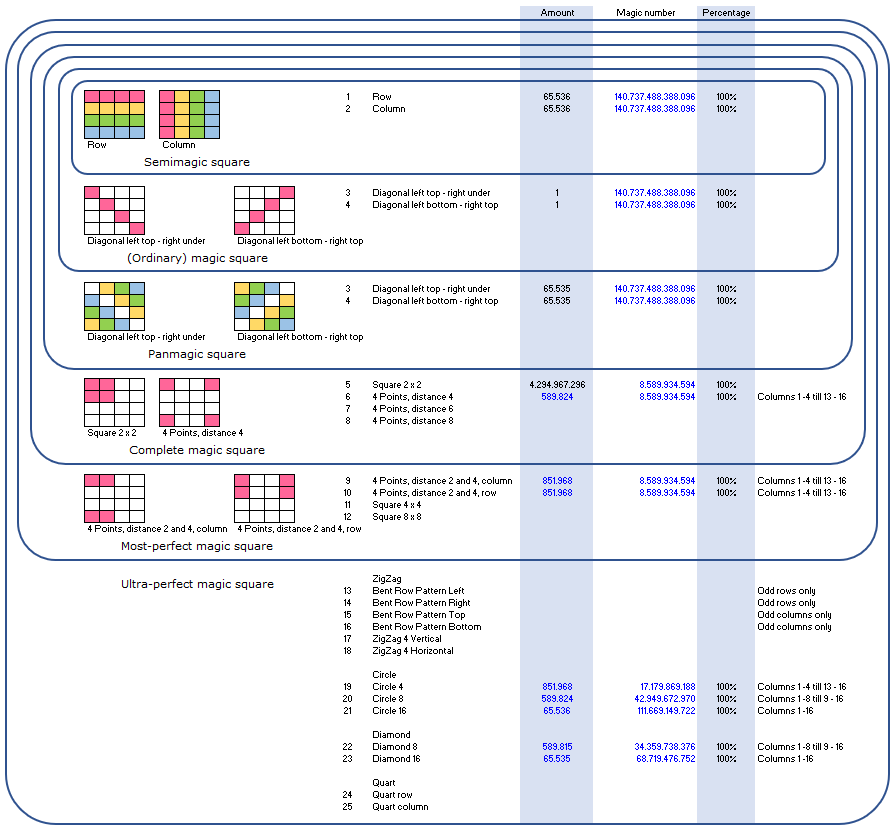Most-perfect magic squares have due to their extreme symmetries excellent qualities to consider the use in encrypting and creating keys. A most-perfect magic square is a magic square were amongst other symmetries also 2x2 subsquares sums to a magic constant.

Below two examples of a 16x16 most-perfect magic square and a 65.536 x 65.536 most-perfect magic square are presented. The method we developed guarantees the creation of a large amount (10^6) of different 16 x 16 most-perfect magic squares. With the same technique we also developed 256 x 256 and 65.536 x 65.526 most-perfect magic squares. Our ambition is to create a 4.294.967.296 x 4.294.967.296 most-perfect magic squares, which requires the use of large computers. With the increasing dimensions of the most-perfect magic squares also the amount of available squares is growing more than exponentionally.

Digital locks with most-perfect magic squares

The  highly symmetric squares can be considered as solution for encryptng and digital locks. Due to the fact, that even the smallest 2 x 2 square within the most-perfect magic square sums to a magic number, this can be a very useful property in creating a new generation of encrypting techniques and digital locks.

Consider for instance the case where two 16 x 16 most-perfect magic squares A and B are added to a new square C. This combined new square satisfies all the symmetry properties. However it is not a most-perfect magic square since the all numbers 1 untill 256 do not appear in this square. If a lock contains a most-perfect magic square A together with the new combined square C, than the key has to be most-perfect magic square B in order to open the lock. A first control can be checking whether all 2 x 2 squares of the key sum to a magic constant. If this is not the case, it's directly clear that a proper digital lock hasn't been used. Other controls could be checking the other symmetries. If the controls satisfy the symmetry checks, then the equation square A + square B = square C is considered. If this last control did work out wel, the lock can be opened.

Two examples of a 16 x 16 most-perfect magic squares

Here are two examples of a most-perfect 16 x 16 magic square we created and where all the 2 x 2 squares sums to the magic constant of 514.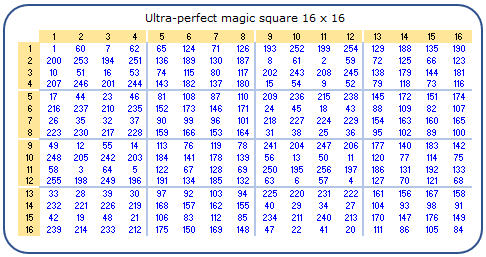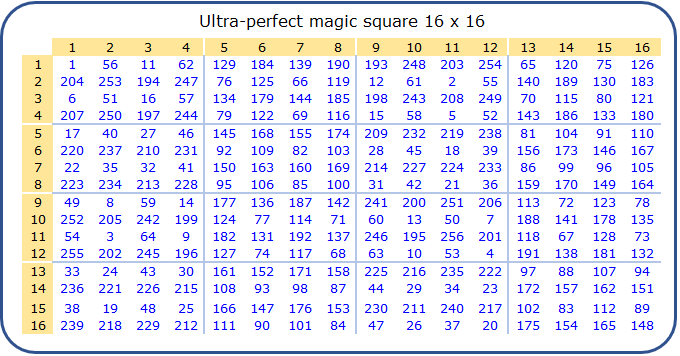Below an overview of the symmetries of these two 16 x 16 most-perfect magic squares is shown. Rows and columns sum to a magic number, properties for a semimagic square. The two diagonals sum to the same magic number, properties for ordinary magic squares. The shifted diagonals sum also to the same magic number which are properties for panmagic squares. And groups of 4 numbers are also summing to a magic number, which are properties of complete magic squares and most-perfect magic squares. And then there are other symmetries as wel, such as bent rows, zig-zag patterns, circles and diamonds.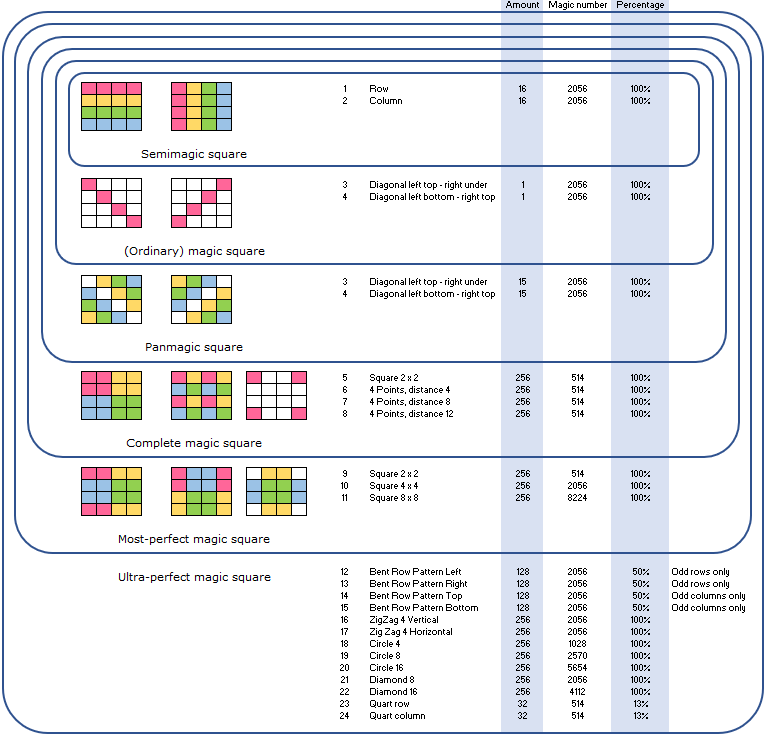Example of a 256 x 256 most-perfect magic squares

Below an overview of the symmetries of a 256 x 256 most-perfect magic squares is shown. Again rows and columns sum to a magic number, properties for a semimagic square. Also the two diagonals sum to the same magic number, properties for ordinary magic squares. The shifted diagonals sum also to the same magic number which are properties for panmagic squares. And groups of 4 numbers are also summing to a magic number, which are properties of complete magic squares and most-perfect magic squares. The most-perfect 256 x 256 magic square together with the control of the symmetry properties shown below can be downloaded (256 x 256 - Control.xlsx).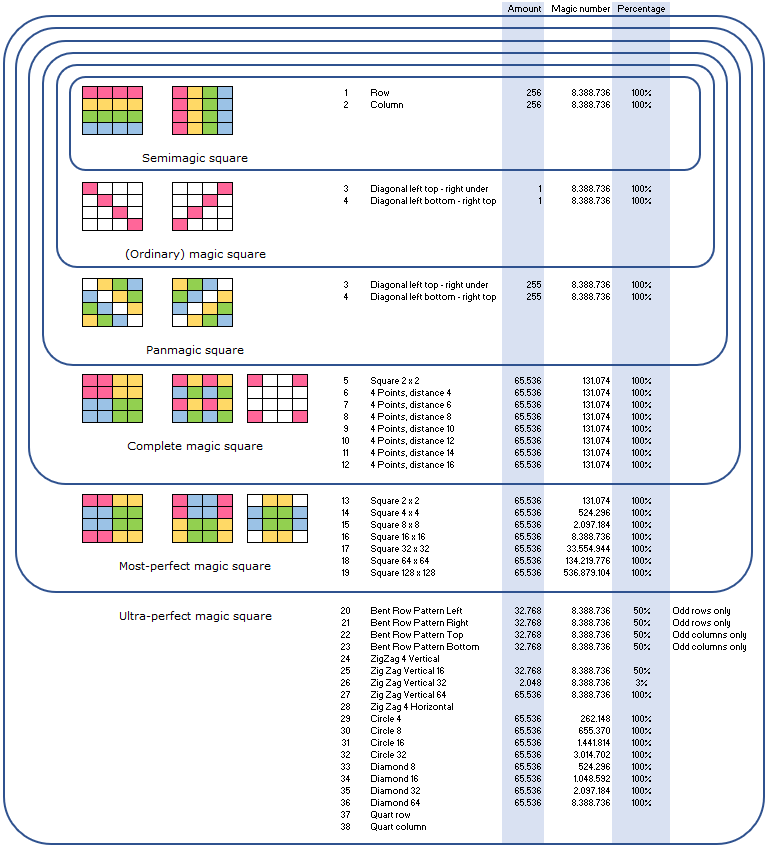Example of a 65.536 x 65.536 most-perfect magic squares

Below an overview of the symmetries of a 65.536 x 65.536 most-perfect magic squares is shown. Again rows and columns sum to a magic number, properties for a semimagic square. Also the two diagonals sum to the same magic number, properties for ordinary magic squares. The shifted diagonals sum also to the same magic number which are properties for panmagic squares. And groups of 4 numbers are also summing to a magic number, which are properties of complete magic squares and most-perfect magic squares. A lot of symmetries have not been investigated, but are expected to be enclosed. The most-perfect 65.536 x 65.536 magic square cannot be downloaded yet, due to it's filesize (33 GB). A file to control the symmetry properties as shown below (65536 x 65536 - Control.xlsm) can be downloaded and also contains the first 16 columns (with row 1 to 65.536) of the magic square.